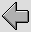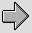Before analysing the (possible) effects of bi-wiring we need to establish a suitable simplified model of the loudspeaker. One of the simplest possible arrangements is illustrated in Figure 4.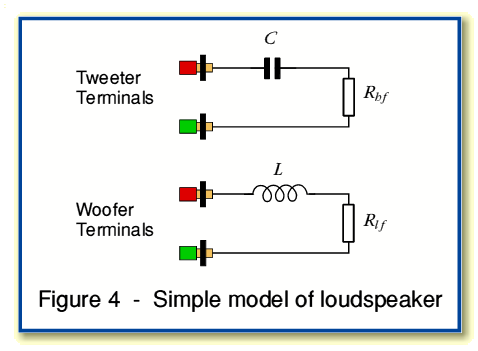In this model the cross-over (filter) networks are deliberately assumed to be about as simple as possible. A series inductor,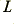, is uses to prevent high frequencies from reaching the woofer, and a series capacitor,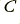, is used to prevent low frequencies from reaching the tweeter. Each actual loudspeaker unit is regarded purely as an effective Radiation Resistance value,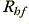and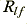. The acoustic power radiated by each unit is then assumed to be proportional to the square of the current through its radiation resistance value. (i.e. the sound pressure produced by each is assumed to be proportional to the current.)

In reality, any practical speaker will have much more complicated properties. However since we are only concerned with seeing if it is possible for bi-wiring to make a change, we can use a simple model of this type. It is, however, important to bear in mind that this means we must interpret any results of our analysis with caution. This is because – even if we conclude that a change may occur – our results cannot be taken as a guide to what level or form such changes may take in a more realistic situation. Hence we are only addressing the “in principle” question of seeing if a change might arise due to bi-wiring. A more detailed and case-specific analysis would be required to assess whether any changes which might arise in a given case were of any audible significance.

For convenience we can assume that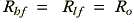, where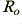is a conveniently chosen standard resistance value. Each of the crossover/unit combinations will have a turn-over frequency set by the chosen values. This will determine the frequency range within which each part of the loudspeaker is responsible for ensuring signals are audible. Again, for simplicity and convenience we can assume these have been set to both equal the same value,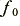. Hence we can say that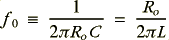The input impedance ,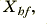of the tweeter, and the input impedance,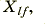of the woofer will therefore be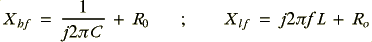Before considering the effects of amplifier-speaker cabling we can now determine the inherent properties of the loudspeaker. Firstly, when its units are used in parallel (as in Fig 1) we can say that the loudspeaker’s impedance will be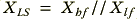where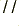represents the parallel combination of the two values.

In this specific, simplified, case it turns out that when we calculate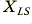it equalsat all frequencies. This result will, of course, not be true in general, so we must interpret any results based upon this particular speaker model with care.

In terms of acoustic output we may say that the pressure radiated by each speaker unit will vary in proportion with the current at each instant through its radiation resistance. We may represent the current through the tweeter as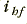and that through the woofer as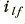. Since the radiation will add coherently we may say that the total sound pressure created will be proportional to the vector total current,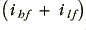. The mean power will therefore be proportional to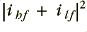, and any phase changes will depend upon the relative phase of the driving signal and the total current.

When using the loudspeaker modelled here we can now represent the situation when employing a single cable (i.e. not bi-wired) by the circuit shown in Figure 5.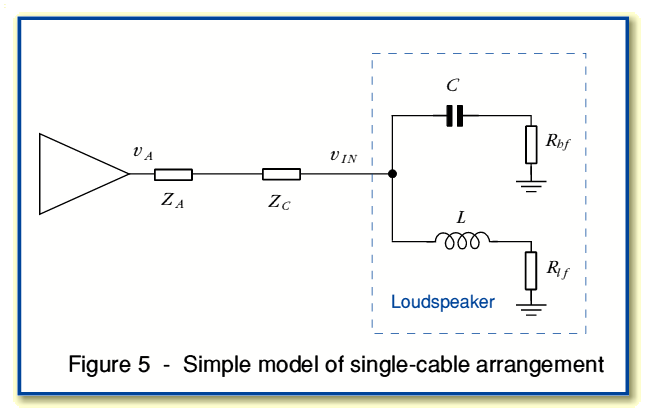Here we can represent the output impedance of the power amplifier as a series impedance,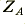, and the impedance of the cable by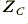. Note thatdoes not represent the characteristic impedance of the cable as a transmission line. It is simply the series resistance and/or inductance of the cables being used.

When the amplifier asserts an output (a.c.) voltage of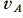this will result in a voltage,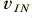, appearing at the loudspeaker terminals. In this simple case we know our loudspeaker has an input impedance (tweeter and woofer sections linked in parallel) of. Hence we can say that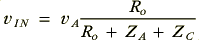In situations where the cable and amplifier impedances are frequency dependent (and not simply resistive) this will lead to a frequency-dependent degree of attenuation, and some relative phase dispersion. However ifandare substantially resistive and independent of frequency the main result is a small reduction in the volume of the resulting sounds. The total load seen by the amplifier will be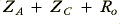at all frequencies in this situation. Hence the power efficiency of the system at all frequencies will be proportional to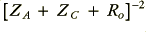.

The circuit is a fairly simple one and has only one current node. This means we can immediately say that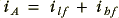where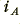is the current being produced by the amplifier at any instant.

The situation when using a bi-wiring arrangement may be represented by the circuit shown in Figure 6. For simplicity it is assumed that the two cables are identical.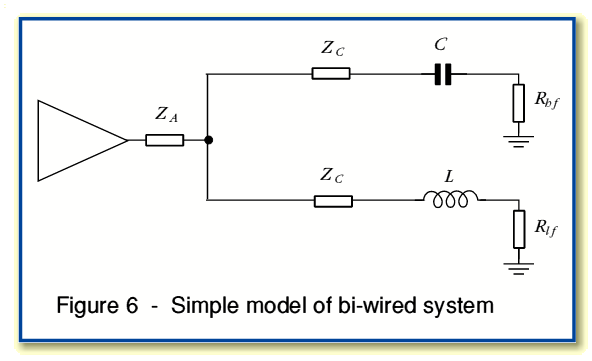As with the previous case, if we assume the cable impedances are essentially resistive then their effect is to increase the total resistance seen in the high-frequency and low-frequency ‘arms’ of the circuit by the same amount. At first sight this seems to imply that we have essentially just altered the overall load by the same amount as before. However this may not be the case as the presence of the separate cable impedances will independently affect the nominal turn-over frequencies of the low-pass and high-pass networks employed. The special case we initially envisaged was that the turn-over frequencies (as well as the load resistances) of the two arms were identical. This meant their parallel combination was purely resistive. However we may now have ‘unbalanced’ the arrangement so that this is not longer true.

As with the single-cable arrangement, the system illustrated in Figure 6 has a single node. Hence we can say that the power efficiency will simply depend upon how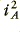may vary with signal frequency. We may therefore look to see if the total load impedance now varies with frequency as if it does, this implies that the above bi-wired arrangement may be expected have a frequency response that differs from that produced with a single cable.